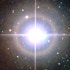BASIC program for visual binary stars

BINARY STARS with short orbital periods can change markedly in appearance from year to year, making descriptions in books out of date. This BASIC program was written by E. R. ‘Ted’ Wood (1914–98), based on equations given by Jean Meeus in his book Astronomical Formulae for Calculators. It can be used to find the separation and position angle of any binary pair whose orbital elements are known, for any required date.

A free BASIC application for Mac, PC, and Linux can be found here.

The program takes as its input the seven orbital elements that describe the orbit of a binary star, as follows:

P .... the orbital period in years

T .... the date of periastron (year and decimals)

a .... the semimajor axis of the orbit, in seconds of arc

e .... the eccentricity of the orbit

i .... the inclination of the orbit to the plane of the sky, in degrees

lower case omega .... argument of periastron, in degrees

upper case omega .... position angle of the ascending node, in degrees

Orbital elements for visual binaries can be found in the Sixth Catalog of Orbits of Visual Binary Stars by Rachel A. Matson, Stephen J. Williams, William I. Hartkopf, and Brian D. Mason. The catalogue is updated regularly.

For a selection of visual binaries suitable for observation with modest telescopes see the table on this page.

PROGRAM

1  REM Binary star orbit program

2  REM By Ted Wood

10 DEF FN C(W) = 0.01745329252*W

20 PX=3.141592654: C=6.283185307

30 INPUT "Period, P (years)                 ";P

40 INPUT "Date of periastron, T             ";T

50 INPUT "Semi-major axis, a                ";A1

60 INPUT "Eccentricity, e                   ";S

70 INPUT "Inclination, i                    ";I

80 INPUT "Arg. of periastron, w             ";W

90 INPUT "P.A. of ascending node            ";L

100 I=FN C(I): L=FN C(L): W=FN C(W)

110 N=C/P

120 INPUT "Date of obs. (year)              ";D

130 MA=N*(D-T)

140 GOSUB 300

150 R=A1-A1*S*COS(EA)

160 Y=SIN(NU+W)*COS(I)

170 X=COS(NU+W)

180 Q=ATN(Y/X)

190 IF X<0 THEN Q=Q+PX: GOTO 210

200 IF Q<0 THEN Q=Q+C

210 TH=Q+L: IF TH>C THEN TH=TH-C

220 RH=R*X/COS(Q)

230 PRINT "P.A. = ";INT(TH/FN C(1)*10+0.5)/10;" deg."

240 PRINT "Sep. = ";INT(RH*100+0.5)/100;" arcsec"

250 INPUT "New date (Y/N) ";AN\$

260 IF AN\$="Y" OR AN\$="y" THEN GOTO 120

265 IF AN\$="N" OR AN\$="n" THEN GOTO 275

270 GOTO 250

275 INPUT "New binary (Y/N) ";AN\$

280 IF AN\$="Y" OR AN\$="y" THEN GOTO 10

290 IF AN\$="N" OR AN\$="n" THEN END

295 GOTO 275

300 M=MA-C*INT(MA/C): EA=M

310 A=EA-(S*SIN(EA))-M

320 IF ABS(A)<0.000001 THEN GOTO 350

330 A=A/(1-(S*COS(EA)))

340 EA=EA-A: GOTO 310

350 TU=SQR((1+S)/(1-S))*TAN(EA/2)

360 NU=2*ATN(TU)

370 RETURN

EXAMPLE

This example shows how the Binary Star Orbit program works. The data are for the star Xi Ursae Majoris. Your computer should duplicate the results given here exactly.

Period, P (years)                 59.88

Date of periastron, T             1995.07

Semi-major axis, a                2.536

Eccentricity, e                   0.398

Inclination, i                    122.13

Arg. of periastron, w             127.94

P.A. of ascending node            101.85

Date of obs. (year)              2010

P.A. = 211.2  deg.

Sep. = 1.61  arcsec

New date (Y/N) Y

Date of obs. (year)              2015.5

P.A. = 174  deg.

Sep. = 1.81  arcsec